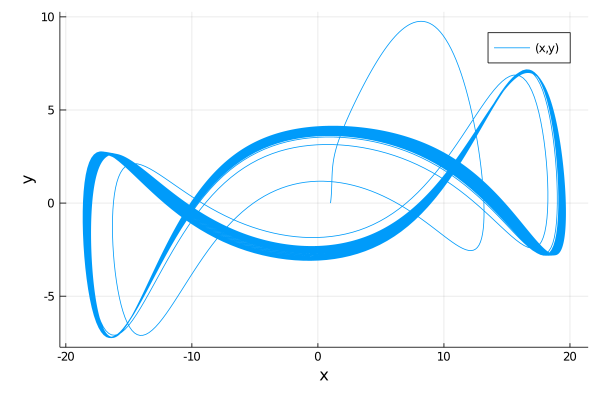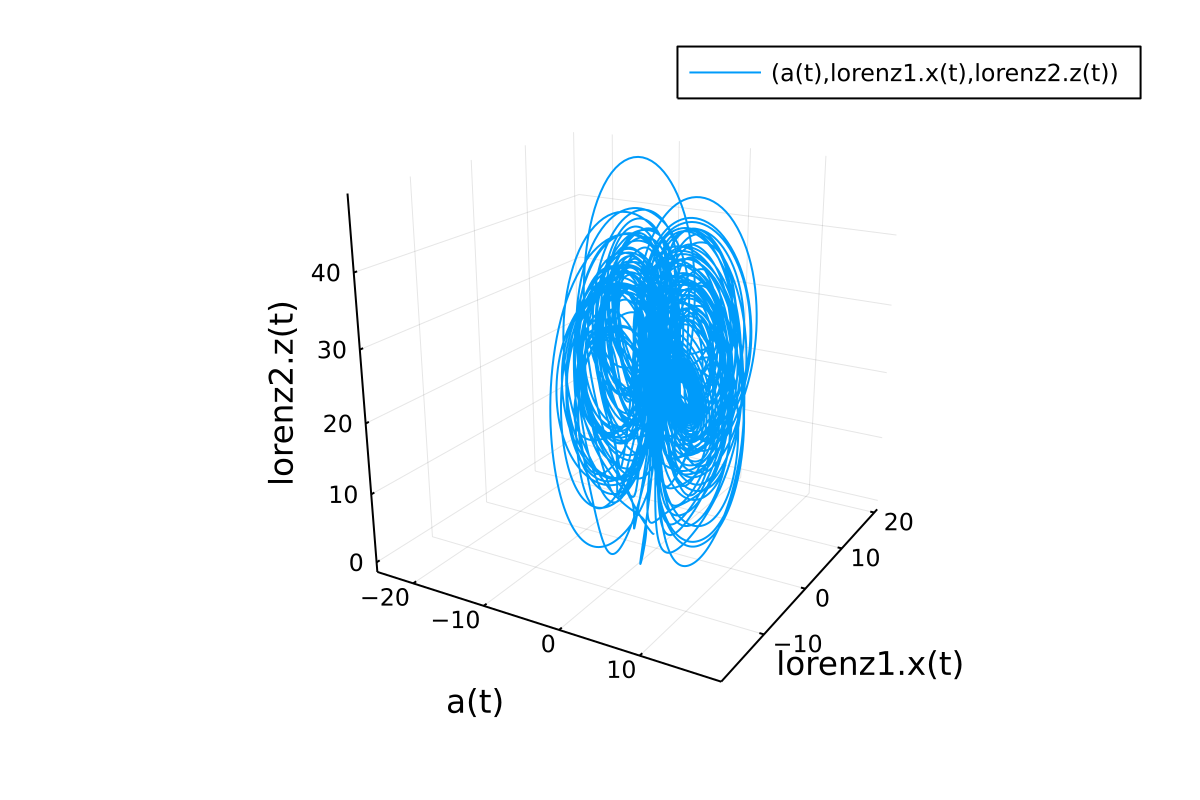## ModelingToolkit.jl

An acausal modeling framework for automatically parallelized scientific machine learning (SciML) in Julia. A computer algebra system for integrated symbolics for physics-informed machine learning and automated transformations of differential equations
Popularity
1212 Stars
Updated Last
7 Months Ago
Started In
February 2018

# ModelingToolkit.jl

ModelingToolkit.jl is a modeling framework for high-performance symbolic-numeric computation in scientific computing and scientific machine learning. It allows for users to give a high-level description of a model for symbolic preprocessing to analyze and enhance the model. ModelingToolkit can automatically generate fast functions for model components like Jacobians and Hessians, along with automatically sparsifying and parallelizing the computations. Automatic transformations, such as index reduction, can be applied to the model to make it easier for numerical solvers to handle.

For information on using the package, see the stable documentation. Use the in-development documentation for the version of the documentation which contains the unreleased features.

## Standard Library

For a standard library of ModelingToolkit components and blocks, check out the ModelingToolkitStandardLibrary

## High-Level Examples

First, let's define a second order riff on the Lorenz equations, symbolically lower it to a first order system, symbolically generate the Jacobian function for the numerical integrator, and solve it.

```using DifferentialEquations, ModelingToolkit

@parameters t σ ρ β
@variables x(t) y(t) z(t)
D = Differential(t)

eqs = [D(D(x)) ~ σ * (y - x),
D(y) ~ x * (ρ - z) - y,
D(z) ~ x * y - β * z]

@named sys = ODESystem(eqs)
sys = structural_simplify(sys)

u0 = [D(x) => 2.0,
x => 1.0,
y => 0.0,
z => 0.0]

p = [σ => 28.0,
ρ => 10.0,
β => 8 / 3]

tspan = (0.0, 100.0)
prob = ODEProblem(sys, u0, tspan, p, jac = true)
sol = solve(prob)
using Plots
plot(sol, idxs = (x, y))```This automatically will have generated fast Jacobian functions, making it more optimized than directly building a function. In addition, we can then use ModelingToolkit to compose multiple ODE subsystems. Now, let's define two interacting Lorenz equations and simulate the resulting Differential-Algebraic Equation (DAE):

```using DifferentialEquations, ModelingToolkit

@parameters t σ ρ β
@variables x(t) y(t) z(t)
D = Differential(t)

eqs = [D(x) ~ σ * (y - x),
D(y) ~ x * (ρ - z) - y,
D(z) ~ x * y - β * z]

@named lorenz1 = ODESystem(eqs)
@named lorenz2 = ODESystem(eqs)

@variables a(t)
@parameters γ
connections = [0 ~ lorenz1.x + lorenz2.y + a * γ]
@named connected = ODESystem(connections, t, [a], [γ], systems = [lorenz1, lorenz2])
sys = structural_simplify(connected)

u0 = [lorenz1.x => 1.0,
lorenz1.y => 0.0,
lorenz1.z => 0.0,
lorenz2.x => 0.0,
lorenz2.y => 1.0,
lorenz2.z => 0.0,
a => 2.0]

p = [lorenz1.σ => 10.0,
lorenz1.ρ => 28.0,
lorenz1.β => 8 / 3,
lorenz2.σ => 10.0,
lorenz2.ρ => 28.0,
lorenz2.β => 8 / 3,
γ => 2.0]

tspan = (0.0, 100.0)
prob = ODEProblem(sys, u0, tspan, p)
sol = solve(prob)

using Plots
plot(sol, idxs = (a, lorenz1.x, lorenz2.z))```# Citation

``````@misc{ma2021modelingtoolkit,
title={ModelingToolkit: A Composable Graph Transformation System For Equation-Based Modeling},
author={Yingbo Ma and Shashi Gowda and Ranjan Anantharaman and Chris Laughman and Viral Shah and Chris Rackauckas},
year={2021},
eprint={2103.05244},
archivePrefix={arXiv},
primaryClass={cs.MS}
}
``````

### Required Packages

View all packages

### Used By Packages

View all packages